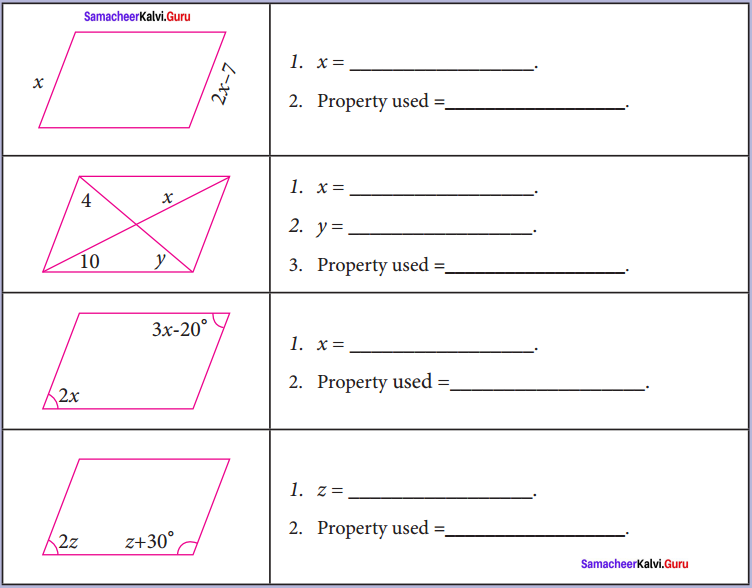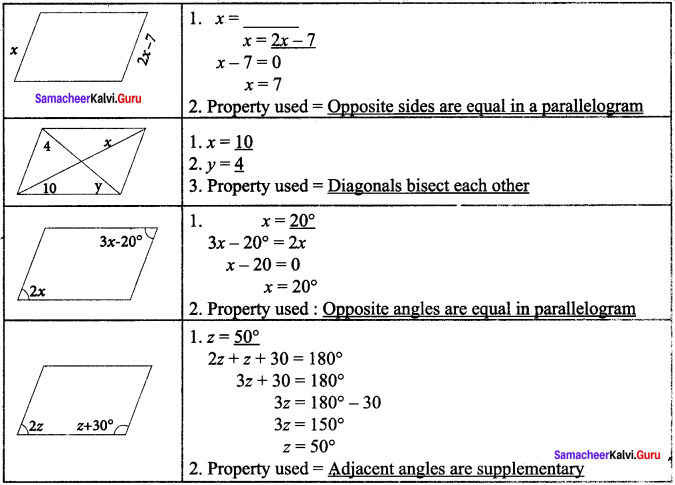Students can Download Maths Chapter 3 Geometry Intext Questions and Answers, Notes Pdf, Samacheer Kalvi 8th Maths Book Solutions Guide Pdf helps you to revise the complete Tamilnadu State Board New Syllabus and score more marks in your examinations.

## Tamilnadu Samacheer Kalvi 8th Maths Solutions Term 2 Chapter 3 Geometry Intext Questions

Exercise 3.1
Try This (Text book Page No. 60)

Question 1.
How will you prove ∆ ABC ~ AD AC?
Proof:
In ∆ ABC & ∆ DAC, ∠C is common and ∠BAC = ∠ADC = 90°
Therefore ∆ ABC ~ AD AC (AA similarity)

Try This (Text book Page No. 61)Question 1.
Check whether the following are Pythagorean triplets

1. 57, 176, 185
2. 264, 265, 23
3. 8, 41, 40

Solution:
1. For Pythagorean triplet, the sum of the squares of 2 sides is equivalent to square of 3rd side (hypotenuse)
Let us check of
572 + 1762 whether = 1852 or not
572 = 3249
1762 = 30976
1852 = 34225
572 + 1762 = 3249 + 30976 = 34225 = 1852
∴ 57, 176 & 185 are Pythagorean triplet.

2. 23, 264, 265
232 = 529
2642 = 69696
232 + 2642 = 70225
2652 = 70225
∴ 232 + 2642 = 2652
∴ Pythagorean triplet

3. 8, 41, 40
82 = 64
402 = 1600
82 + 402 = 1664
412 = 1681
82 + 402 ≠ 412 = 1
∴ They are not Pythagorean triplet

Activity – 1 (Text book Page No. 62)

We can construct sets of Pythagorean triplets as follows. Let m and n be any two positive integers (m > n):
(a, b, c) is a Pythagorean triple if a = m2 – n2, b = 2mn and c = m2 + n2 (Think, why?) Complete the table.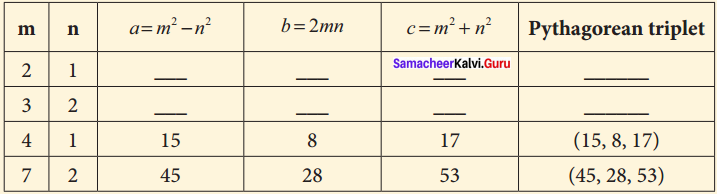Solution: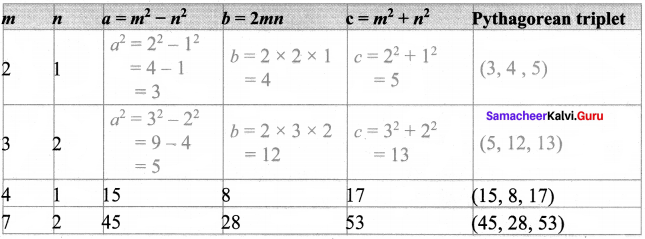Activity – 2 (Text book Page No. 65)

Question 1.
Find all integer-sided right angled triangles with hypotenuse 85
Solution:
(x + y)2 – 2xy = 852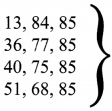Pythagorean triplets with hypotenuse 85.

Exercise 3.3
Try These (Text book Page No. 68)

Question 1.
The area of the trepezium is ……..
$$\frac{1}{2}$$ x h x (a + b) sq. units!Question 2.
The distance between the parallel sides of a trapezium is called as ………
its height

Question 3.
If the height and parallel sides of a trapezium are 5 cm, 7 cm and 5 cm respectively, then its area is ……..
30 sq cm
Hint:
= $$\frac{1}{2}$$ x h x (a + b) sq. units
= $$\frac{1}{2}$$ x 5 x (7 + 5)
= $$\frac{1}{2}$$ x 5 x 12 = 30 sq.cm

Question 4.
In an isosceles trapezium, the non-parallel sides are ……….. in length.
Equal.

Question 5.
To construct a trapezium, ………… measurements are enough.
Four.

Question 6.
If the area and sum of the parallel sides are 60 cm2 and 12 cm, its height is ………..
10 cm
Hint:
Area of the trapezium = $$\frac{1}{2}$$ x h (a + b)
60 = $$\frac{1}{2}$$ x h x (12)
h = $$\frac{60×2}{12}$$ = 10 cm

Exercise 3.4
Think (Text book Page No. 74)Question 1.
Can a rhombus, a square or a rectangle be called as a parallelogram? Justify your answer.
Solution:
Yes, a rhombus, a square or a rectangle can be called as parallelogram as the opposite sides are equal and parallel and diagonals bisect each other in this figures.

Try These (Text book Page No. 74)

Question 1.
In a parallelogram, the opposite sides are …….. and ……….
equal, parallel

Question 2.
If ∠A of a parallelogram ABCD is 100° then, find ∠B, ∠C and ∠D .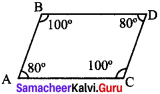∠B = 80°
∠C = 100°
∠D = 80°

Question 3.
Diagonals of a parallelogram each other.
BisectQuestion 4.
If the base and height of the parallelogram are 20 cm and 5 cm then, its area is ………